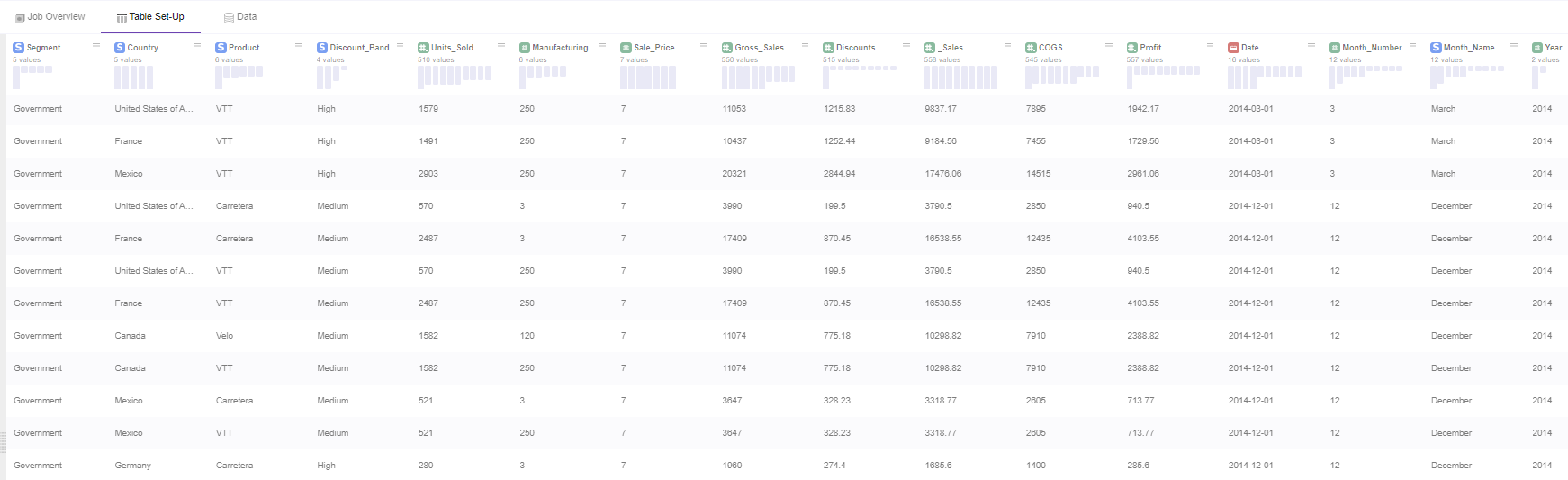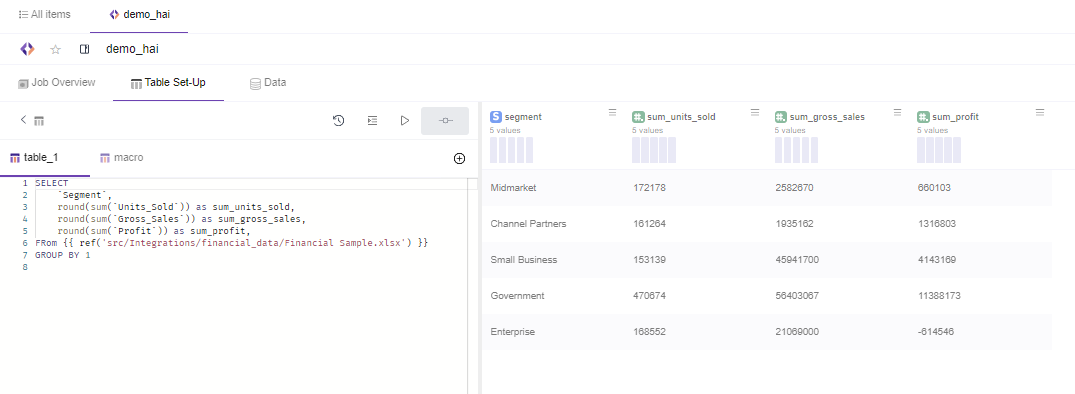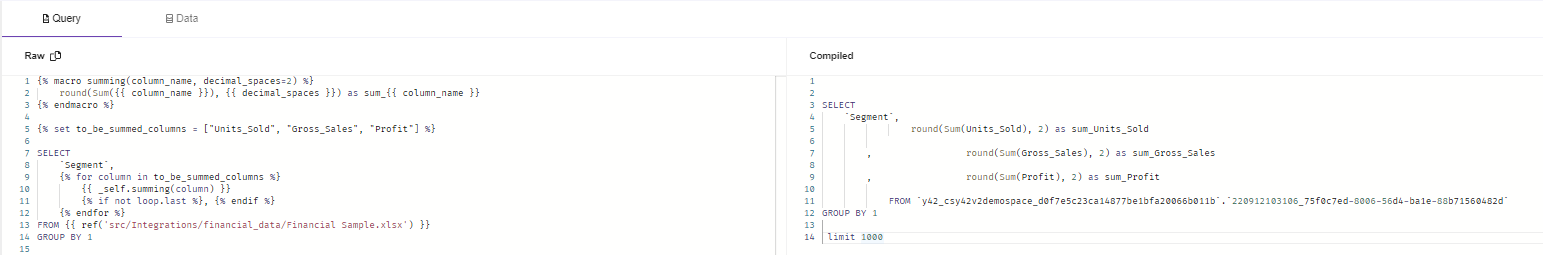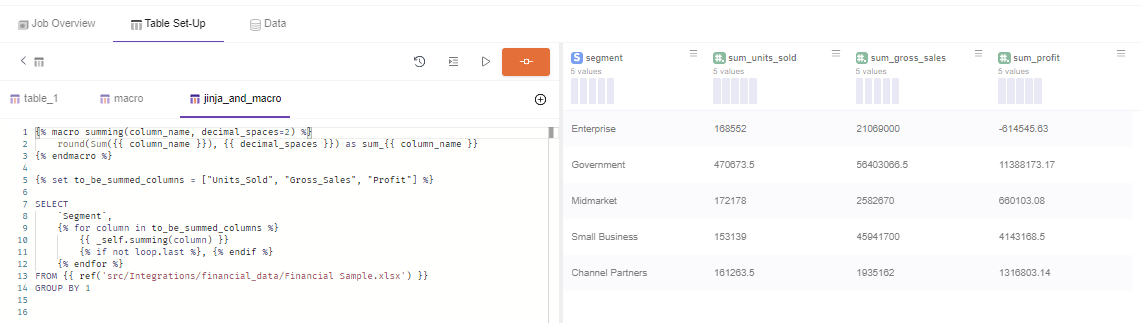Writing SQL statement is sometimes a challenge not only for the difficulty in writing them but also for the repetitive parts, which make the process even more painful and prone to errors.

SQL Models in Y42 has integrated Jinja templating language in its editor, making your SQL statements more powerful. Within Jinja you can use macros, which should be considered like functions, making the repetitive pieces of SQL code programmatic.

## Jinja Syntax & Capabilities

With Jinja you can use your SQL statements in Y42 SQL model programmatically making the code shorter and reusable.

To name some of the features ,with Jinja you can:

• Use `if` statements and `for` loops in SQL

You can do so by using Statements `{% statement goes here %}`: Statements are used for control flow like if statement and for loops, define macros or set Variables .

• Reference Variables or call Macros ** via Expressions** `{{ expression goes here }}`

Expressions are used when you want to output a string. You can use expressions to reference variables and call macros.

• Add Comments `{# Comment goes here #}.`

Jinja comments are used to add notes inside the code which will be ignored during the compilation.

## Getting started​ with an example​

Let's add a typical use case scenario.

We have this Input data:And we want to sum up and round, the columns `Units_Sold, Gross_Sales, Profit`, grouped by 'Segment' for your organization.

The typical SQL statement would be:

``````SELECT
`Segment`,
round(sum(`Units_Sold`)) as sum_units_sold,
round(sum(`Gross_Sales`)) as sum_gross_sales,
round(sum(`Profit`)) as sum_profit,
FRom {{ ref('src/Integrations/financial_data/Financial Sample.xlsx') }}
GROUP BY 1
``````

The result would be:This approach works works well if we want to sum and round a small amount of columns.

Imagine if you have a hundred columns, adding the statements for each is very boring, takes time and you get prone to errors while doing it.

Here is where Jinja + Macros come to the rescue:

``````{% macro summing(column_name, decimal_spaces=2) %}
round(Sum({{ column_name }}), {{ decimal_spaces }}) as sum_{{ column_name }}
{% endmacro %}

{% set to_be_summed_columns = ["Units_Sold", "Gross_Sales", "Profit"] %}

SELECT
`Segment`,
{% for column in to_be_summed_columns %}
{{ _self.summing(column) }}
{% if not loop.last %}, {% endif %}
{% endfor %}
FROM {{ ref('src/Integrations/financial_data/Financial Sample.xlsx') }}
GROUP BY 1
``````

As you can see, when compiled it is the same query as the previous one, and it will have the same result set.The only difference is that it is more scalable, because if you need to add more columns you simply need to add the column names in the variable list.

{% set to_be_summed_columns = ["Units_Sold", "Gross_Sales", "Profit"] %}## Step by step process

### 1- Notice the repetitive piece of SQL.

``````SELECT
`Segment`,
round(sum(`Units_Sold`)) as sum_units_sold,
round(sum(`Gross_Sales`)) as sum_gross_sales,
round(sum(`Profit`)) as sum_profit,
FRom {{ ref('src/Integrations/financial_data/Financial Sample.xlsx') }}
GROUP BY 1
``````

As you can see in the pure SQL statement in the example the functions are repetitive.

### 2- Use a for loop in the Select statement.

The repetitive functions can be emulated using a for loop.

{% for column in tobe_summed_columns %} → _Opens the for loop

{% endfor %} → Ends the for loop

``````SELECT
`Segment`,
{% for column in to_be_summed_columns %}
{{ _self.summing(column) }}
{% if not loop.last %}, {% endif %}
{% endfor %}
FROM {{ ref('src/Integrations/financial_data/Financial Sample.xlsx') }}
GROUP BY 1
``````

### 3- Set Variables.

Now you need to set the variable that will be used in the for loop. You can do that using the command "set".

In our case we add the list of the columns that will be used in the Sum and Round function.

``````{% set to_be_summed_columns = ["Units_Sold", "Gross_Sales", "Profit"] %}
``````

Here the "function" will be written. In our case the function name is summing() and has two parameters " column_name, decimal_spaces=2 "

Notice that we use variables {{ }} to output the parameters inside the function.

``````{% macro summing(column_name, decimal_spaces=2) %}
round(Sum({{ column_name }}), {{ decimal_spaces }}) as sum_{{ column_name }}
{% endmacro %}
``````

### 5- Call the Macro inside the Select statement.

You can call the macro using:

{{ _self.name_of_the_macro(Parameter) }}

In our case:

`````` {{ _self.summing(column) }}
``````

Note: we have already added it in the step 2 spinet inside the for loop, for the sake of simplicity.

### 6- Add the commas between the columns in the SQL statement

In order for the columns to be separated you need to separate them with commas.

You can do it via an if statement and loop.last function combination:

``````{% if not loop.last %}
,
{% endif %}
``````

As you can notice we add a comma at the end of the column until the last loop so we have a valid SQL statement.

This is how we arrive to the complete statement in the example

``````{% macro summing(column_name, decimal_spaces=2) %}
round(Sum({{ column_name }}), {{ decimal_spaces }}) as sum_{{ column_name }}
{% endmacro %}

{% set to_be_summed_columns = ["Units_Sold", "Gross_Sales", "Profit"] %}

SELECT
`Segment`,
{% for column in to_be_summed_columns %}
{{ _self.summing(column) }}
{% if not loop.last %}, {% endif %}
{% endfor %}
FROM {{ ref('src/Integrations/financial_data/Financial Sample.xlsx') }}
GROUP BY 1
``````